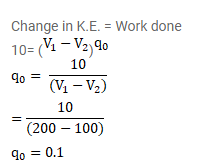# The kinetic energy of a charged particle decreases byQuestion:

The kinetic energy of a charged particle decreases by $10 \mathrm{~J}$ as it moves from a point at potential $100 \mathrm{~V}$ to a point at potential $200 \mathrm{~V}$. Find the charge on the particle.

Solution: